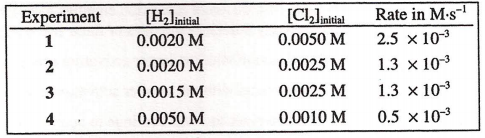# Problem: Initial rate data for the reaction 2 H2 (g) + Cl2 (g) are given in the table. What is the rate law for this reaction? (A) rate k [Cl2]2 (B) rate = k [Cl2] (C) rate = k [H2] (D) rate = k [H2] [Cl2]

###### FREE Expert Solution
86% (352 ratings)
###### Problem Details

Initial rate data for the reaction 2 H(g) + Cl(g) are given in the table. What is the rate law for this reaction?

(A) rate k [Cl2]2

(B) rate = k [Cl2]

(C) rate = k [H2]

(D) rate = k [H2] [Cl2]Frequently Asked Questions

What scientific concept do you need to know in order to solve this problem?

Our tutors have indicated that to solve this problem you will need to apply the Rate Law concept. You can view video lessons to learn Rate Law. Or if you need more Rate Law practice, you can also practice Rate Law practice problems.

What is the difficulty of this problem?

Our tutors rated the difficulty ofInitial rate data for the reaction 2 H2 (g) + Cl2 (g) are gi...as medium difficulty.

How long does this problem take to solve?

Our expert Chemistry tutor, Sabrina took 6 minutes and 30 seconds to solve this problem. You can follow their steps in the video explanation above.

What professor is this problem relevant for?

Based on our data, we think this problem is relevant for Professor Kulatunga's class at USF.